Question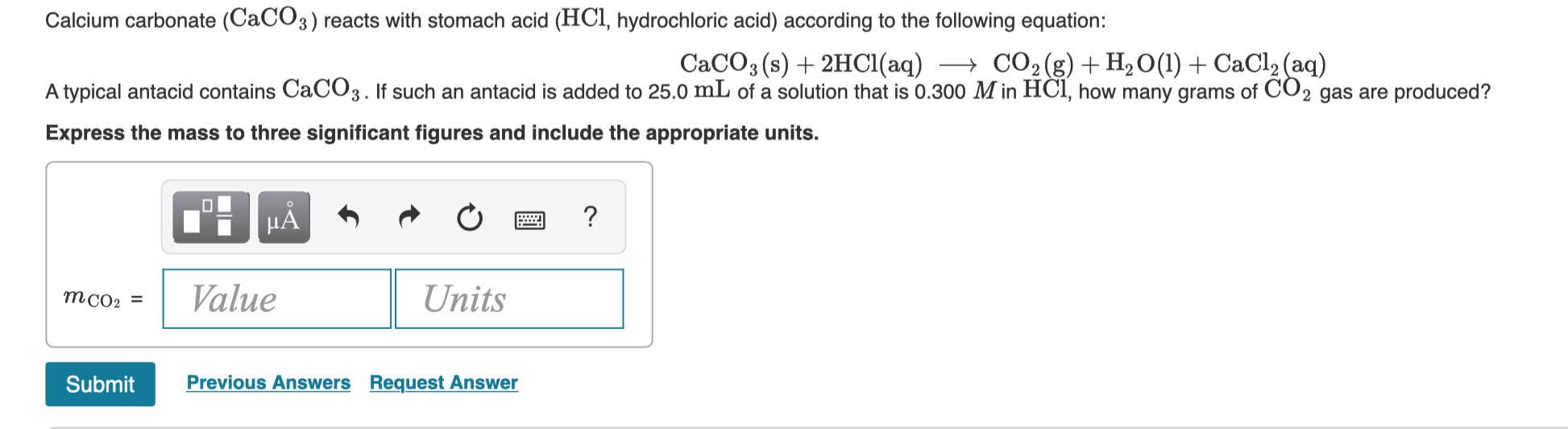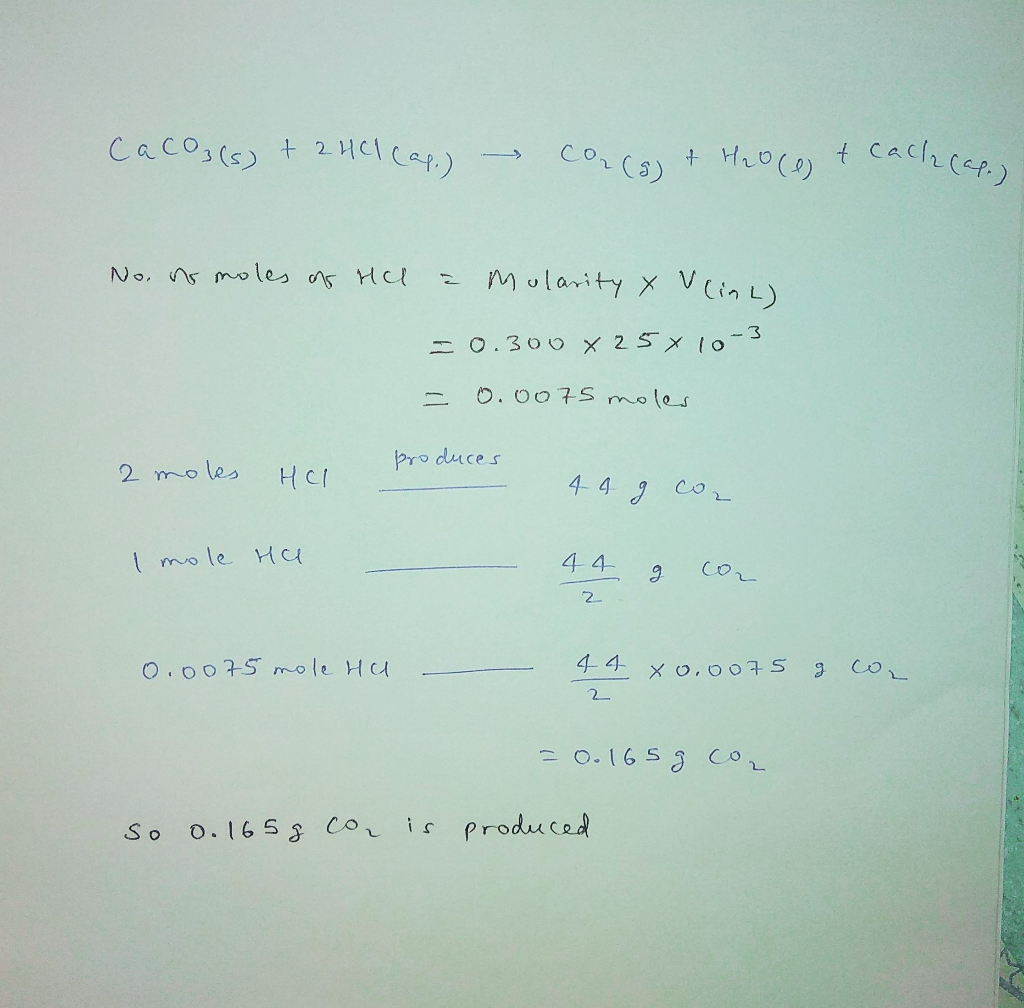#### Earn Coins

Coins can be redeemed for fabulous gifts.

Similar Homework Help Questions
• ### Calcium carbonate, CaCO3, reacts with stomach acid, (HCI, hydrochloric acid) according to the following equation: CaCO3(s)...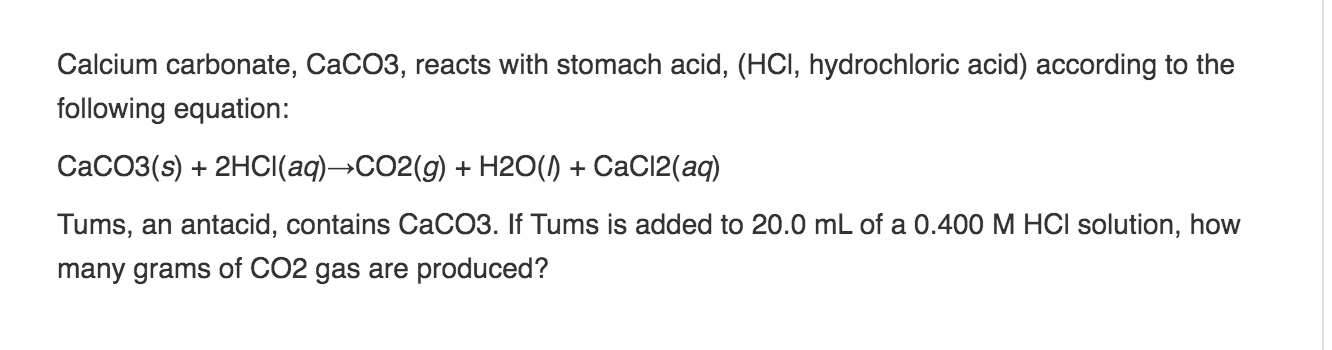Calcium carbonate, CaCO3, reacts with stomach acid, (HCI, hydrochloric acid) according to the following equation: CaCO3(s) + 2HCl(aq)-CO2(g) + H2O(1) +CaCl2(aq) Tums, an antacid, contains CaCO3. If Tums is added to 20.0 mL of a 0.400 M HCl solution, how many grams of CO2 gas are produced?

• ### Calcium carbonate (CaCO3) reacts with stomach acid (HCl, hydrochloric acid) according to the following equation: CaCO3(s)+2HCl(aq)⟶CO2(g)+H2O(l)+CaCl2(aq)...

Calcium carbonate (CaCO3) reacts with stomach acid (HCl, hydrochloric acid) according to the following equation: CaCO3(s)+2HCl(aq)⟶CO2(g)+H2O(l)+CaCl2(aq) Tums, an antacid, contains CaCO3. If Tums is added to 10.0 mL of a solution that is 0.400 M in HCl, how many grams of CO2 gas are produced?

• ### Calcium carbonate is used in many antacids to neutralize stomach acid, as depicted by the following...

Calcium carbonate is used in many antacids to neutralize stomach acid, as depicted by the following chemical equation: CaCO3(s) + 2 HCl(aq) → CaCl2 + H2O(l) + CO2(g) If 250. mg of calcium carbonate reacts with 5.64 g of hydrochloric acid, how much hydrochloric acid will remain? Provide your answer in g.

• ### When calcium carbonate is added to hydrochloric acid, calcium chloride, carbon dioxide, and water are produced....

When calcium carbonate is added to hydrochloric acid, calcium chloride, carbon dioxide, and water are produced. CaCO3(s)+2HCl(aq)⟶CaCl2(aq)+H2O(l)+CO2(g) How many grams of calcium chloride will be produced when 28.0 g of calcium carbonate is combined with 13.0 g of hydrochloric acid? mass of CaCl2 : g Which reactant is in excess? HCl CaCO3 CaCO 3 How many grams of the excess reactant will remain after the reaction is complete? mass of excess reactant:

• ### Part A Calcium carbonate (CaCOs) reacts with stomach acid (HCI hydrochionic acid) according to the following...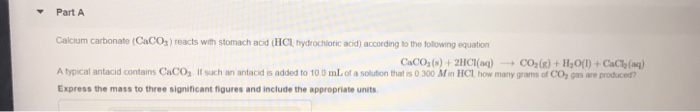Part A Calcium carbonate (CaCOs) reacts with stomach acid (HCI hydrochionic acid) according to the following equation Atypical antacid contains CaCO, ll sch an antacid is added to 100mLofasou ton that s ? 300 Mnila how many ga satch gas ae poacen CaCO, (s) + 2HCl(aq)-+ CO,(g) + F,O() + CaCh(aq) Express the mass to three significant figures and include the appropriate units

• ### When calcium carbonate is added to hydrochloric acid, calcium chloride, carbon dioxide, and water are produced....

When calcium carbonate is added to hydrochloric acid, calcium chloride, carbon dioxide, and water are produced. CaCO3(s)+2HCl(aq)⟶CaCl2(aq)+H2O(l)+CO2(g) How many grams of calcium chloride will be produced when 30.0 g of calcium carbonate is combined with 14.0 g of hydrochloric acid?

• ### When calcium carbonate is added to hydrochloric acid, calcium chloride, carbon dioxide, and water are produced. CaCO3(s...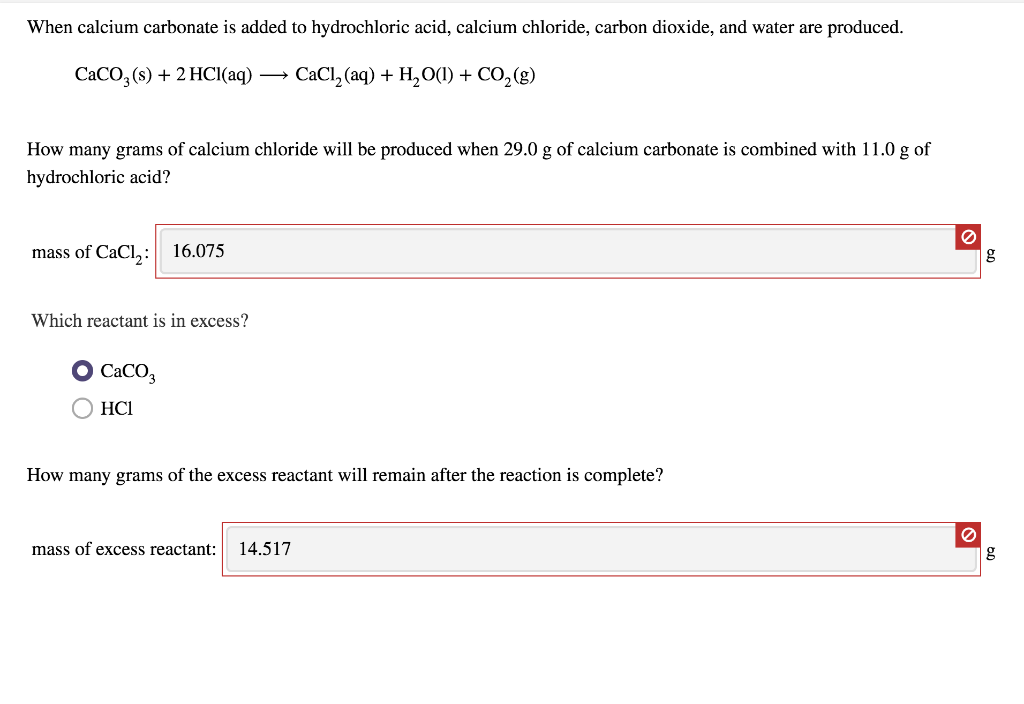When calcium carbonate is added to hydrochloric acid, calcium chloride, carbon dioxide, and water are produced. CaCO3(s) + 2 HCl(aq) → CaCl2 (aq) + H2O(1) + CO2(8) How many grams of calcium chloride will be produced when 29.0 g of calcium carbonate is combined with 11.0 g of hydrochloric acid? mass of CaCl,: 16.075 g Which reactant is in excess? Caco OHCI How many grams of the excess reactant will remain after the reaction is complete? mass of excess reactant:...

• ### When calcium carbonate is added to hydrochloric acid, calcium chloride, carbon dioxide, and water are produc CaCO3(...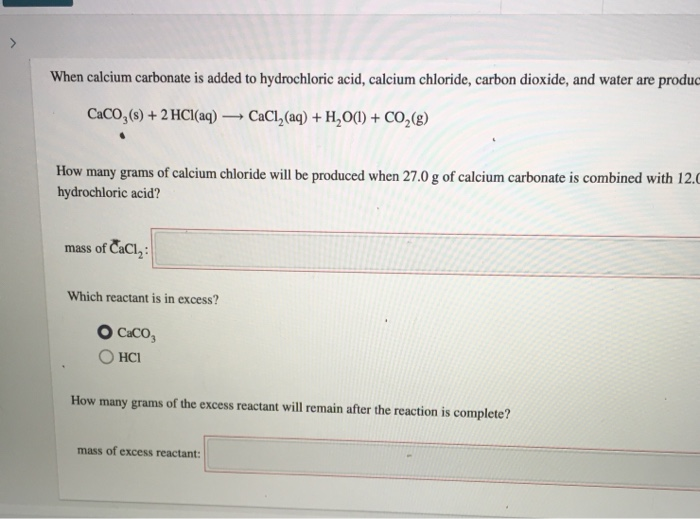When calcium carbonate is added to hydrochloric acid, calcium chloride, carbon dioxide, and water are produc CaCO3(s) + 2 HCl(aq) CaCl (aq) + H2O(l) + CO2(g) How many grams of calcium chloride will be produced when 27.0 g of calcium carbonate is combined with 12. hydrochloric acid? mass of CaCl2: Which reactant is in excess? O Caco, OHCI How many grams of the excess reactant will remain after the reaction is complete? mass of excess reactant:

• ### When calcium carbonate is added to hydrochloric acid, calcium chloride, carbon dioxide, and water are produced....

When calcium carbonate is added to hydrochloric acid, calcium chloride, carbon dioxide, and water are produced. CaCO3(s)+2HCl(aq)⟶CaCl2(aq)+H2O(l)+CO2(g) How many grams of calcium chloride will be produced when 31.0 g of calcium carbonate is combined with 15.0 g of hydrochloric acid? How many grams of the excess reactant will remain after the reaction is complete?

• ### When calcium carbonate is added to hydrochloric acid, calcium chloride, carbon dioxide, and water are produced....

When calcium carbonate is added to hydrochloric acid, calcium chloride, carbon dioxide, and water are produced. CaCO3(s)+2HCl(aq)⟶CaCl2(aq)+H2O(l)+CO2(g) How many grams of calcium chloride will be produced when 30.0 g of calcium carbonate is combined with 12.0 g of hydrochloric acid? mass of CaCl2: 18.25g How many grams of the excess reactant will remain after the reaction is complete? mass of excess reactant: g